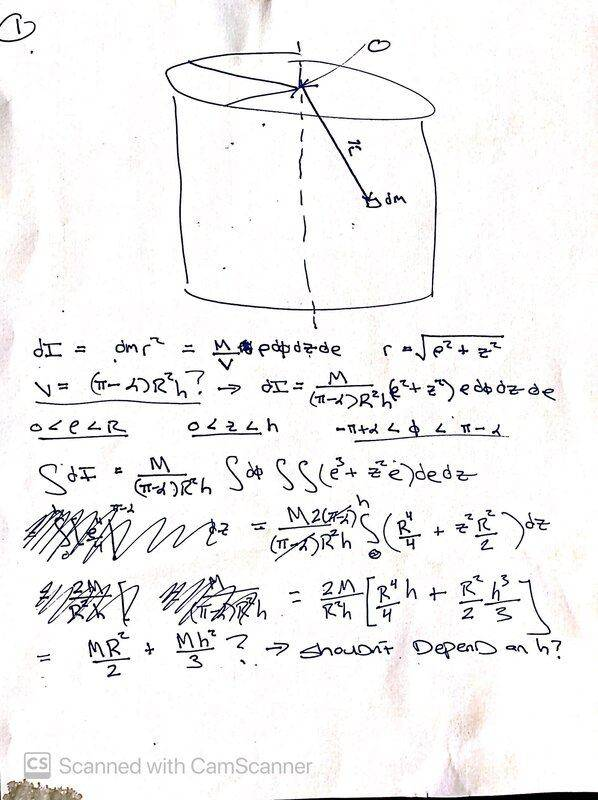# Moment of Inertia of a Solid Cylinder With a Wedge Removed

cedoty1989
Homework Statement:
Imagine a solid cylinder height h able to rotate vertically around z-axis (centered at x=0 y=0). There is a wedge cut out so that when looking down with z hat pointing out of the page there is an angle 2a formed such that their is symmetry with respect to reflection over the x axis (See picture). The question is to calculate the moment of inertia.
Relevant Equations:
I=∫∫∫ dm r^2 -> Cylindrical Coords: (r from 0 to R) (z from zero to h) (theta from -Pi + a to Pi-a)

Uniform density -> dm=(M/V)dV

dV = (dr)r(dtheta)(dz)

The rest of the equations are in the picture...